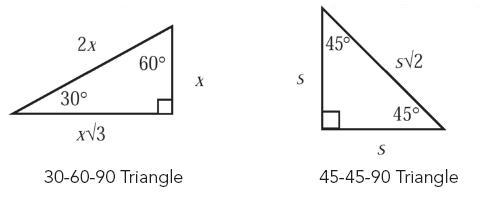## Monday, January 12, 2015

### 25 Math Concepts You Absolutely Need to Know

•  The area of a circle is A = πr2, where r is the radius of the circle.
•  The circumference of a circle is C = 2πr, where r is the radius of the circle. The circumference can
also be expressed as πd, because the diameter is always twice the radius.
•  The area of a rectangle is A = lw, where l is the length of the rectangle and w is the width of the
rectangle.
•  The area of a triangle is A = ½bh, where b is the base of the triangle and h is the height of the
triangle.
•  The volume of a rectangular prism is V = lwh, where l is the length of the rectangular prism, w is
the width of the rectangular prism, and h is height of the rectangular prism.
•  The volume of a cylinder is V = πr2h, where r is the radius of one of the bases of the cylinder and
h is the height of the cylinder.
•  The perimeter is the distance around any object.
•  The Pythagorean Theorem only applies to right angles and states that c2 = a2 + b2, where c is the hypotenuse of the triangle and
a and b are two sides of the triangle.
•  The following are angle measures and side lengths for Special Right Triangles:•  In an equilateral triangle, all three sides have the same length, and each of the angles equals 60°.
•  In an isosceles triangle, two sides have the same length, and the angles opposite those sides are
congruent.
•  The complete arc of a circle has 360°.
•  A straight line has 180°.
•  A prime number is any number that can only be divided by itself and 1.
•  Squaring a negative number yields a positive result.
•  To change any fraction to a decimal, divide the numerator by the denominator.
•  If two numbers have one or more divisors in common, those are the common factors of the
numbers.
•  To calculate the mean, or average, of a list of values, divide the sum of the values by the number
of values in the list.
•  The median is the middle value of a list of numbers that is in either ascending or descending
order.
•  The mode is the value that appears the greatest number of times in a list.
•  A ratio expresses a mathematical comparison between two quantities. (1/4 or 1:4)
•  A proportion is an equation involving two ratios. (1/4 = x/8 or 1:4 = x:8)
•  When multiplying exponential expressions with the same base, add the exponents.
•  When dividing exponential expressions with the same base, subtract the exponents.
•  When raising one power to another power, multiply the exponents.

Source: Benchprep.com

Please visit my other blogs too: http://edwardanil.blogspot.com for information and http://netsell.blogspot.com for internet marketing. Thanks !!